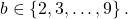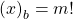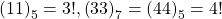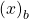# Factorials as repdigits in base b

Nurettin Irmak and Alain Togbé
Notes on Number Theory and Discrete Mathematics
Print ISSN 1310–5132, Online ISSN 2367–8275
Volume 28, 2022, Number 1, Pages 21—25
DOI: 10.7546/nntdm.2022.28.1.21-25

## Details

### Authors and affiliations

Nurettin IrmakDepartment of Fundamental Science, Faculty of Engineering and Natural Science,
Konya Technical University, Konya, Turkey

Alain TogbéDepartment of Mathematics, Purdue University Northwest
1401 S. U. S. 421., Westville, IN 46391, United States

### Abstract

LetIn this paper, we show that the solutions of the equationare, wherehas at least two digits.

### Keywords

• Factorials
• Repdigits
• Diophantine equation

• 11D72
• 11A07
• 11B50

### References

1. Adegbindin, C., Luca, F., & Togbé, A. (2019). Lucas numbers as sums of two repdigits. Lithuanian Mathematical Journal, 59, 295–304.
2. Adegbindin, C., Luca, F., & Togbé, A. (2020). Pell and Pell–Lucas numbers as sums of two repdigits. Bulletin of the Malaysian Mathematical Sciences Society, Series 2, 43(2), 1253–1271.
3. Irmak, N. (2019). On factorials in Perrin and Padovan sequences. Turkish Journal of Mathematics, 43, 2602–2609.
4. Irmak, N., & Togbé, A. (2018). On repdigits as product of consecutive Lucas Numbers. Notes on Number Theory and Discrete Mathematics, 24(3), 95–102.
5. Luca, F. (2000). Fibonacci and Lucas numbers with only one distinct digit. Portugaliae Mathematica, 50, 243–254.
6. Luca, F., Normenyo, B. V., & Togbé, A. (2019). Repdigits as sums of four Pell numbers. Boletin de la Sociedad Matematica Mexicana, 25(2), 249–266.
7. Marques, D. (2012). The order of appearance of product of consecutive Fibonacci numbers. Fibonacci Quarterly, 50, 132–139.
8. Marques, D., & Lengyel, T. (2014). The 2-adic Order of the Tribonacci Numbers and the Equation Tn = m!. Journal of Integer Sequences, 17, Article 14.10.1.
9. Marques, D., & Togbé, A. (2012). On repdigits as product of consecutive Fibonacci numbers. Rendiconti dell’Istituto di matematica dell’Universit di Trieste, 44, 393–397.
10. Normenyo, B. V., Luca, F., & Togbé, A. (2018). Repdigits as Sums of Four Fibonacci or Lucas Numbers. Journal of Integer Sequences, 21, Article 18.7.7.
11. Rayaguru, S. G., & Panda, G. K. (2018). Repdigits as products of consecutive balancing or Lucas-balancing numbers. Fibonacci Quarterly, 56(4), 319–324.
12. Rayaguru, S. G., & Panda, G. K. (2021). Repdigits as sums of two associated Pell numbers. Applied Mathematics E-notes, 21, 402–409.
13. Rayaguru, S. G., & Panda, G. K. (2021). Balancing and Lucas-Balancing numbers
expressible as sum of two repdigits. Integers, 21, A7.
14. Siar, Z., & Keskin, R. (2020). Repdigits as sums of two Lucas numbers. Applied Mathematics E-Notes, 20, 33–38.